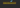# What is the difference between Rest and spread operator## Rest Parameters ( … )

Rest parameters help us to pass an infinite number of function arguments.

(Rest parameters) Example

``````function sum(a,b,...remaining){

console.log(a)

console.log(b)

console.log(remaining)

}

console.log(sum(1,2,3,4,5,6,7))

//a -> 1

//b - > 2

//remaining - > [3,4,5,6,7]``````

In the above code, `sum` function accepts three arguments `a`, `b` and `remaining`.where first two arguments values are `1`,`2` everything we passed after the first two arguments are represented as an array.

Spread operator helps us to expand the strings or array literals or object literals.

### Split the strings

`````` let name = "JavaScript";

let arrayOfStrings = [...name];

console.log(arrayOfStrings);

//Ouptut -> ["J", "a", "v", "a", "S", "c", "r", "i", "p", "t"]``````

It splits the single string into the array of strings

### Combine arrays

``````const group1 = [1,2,3];
const group2 = [4,5,6];

const allGroups = [...group1,...group2];

console.log(allGroups)

//output -> [1, 2, 3, 4, 5, 6]``````

In the above code, we combined `group1` and `group2` by using the spread operator.

Consider we have an array of numbers we need to pass into the function as an arguments.

``````function sum(a,b,c){

return a+b+c;

}

const nums = [1,2,3];

//function call

sum(...nums)

//output -> 6``````

### Summary

• If you see (…) dots on the function calls then it is spread, operator.
• spread operator helps us to create the copies.
• Spread operator helps us to Combine Arrays.
• if you see (…) on the function parameters then it is rest parameters.